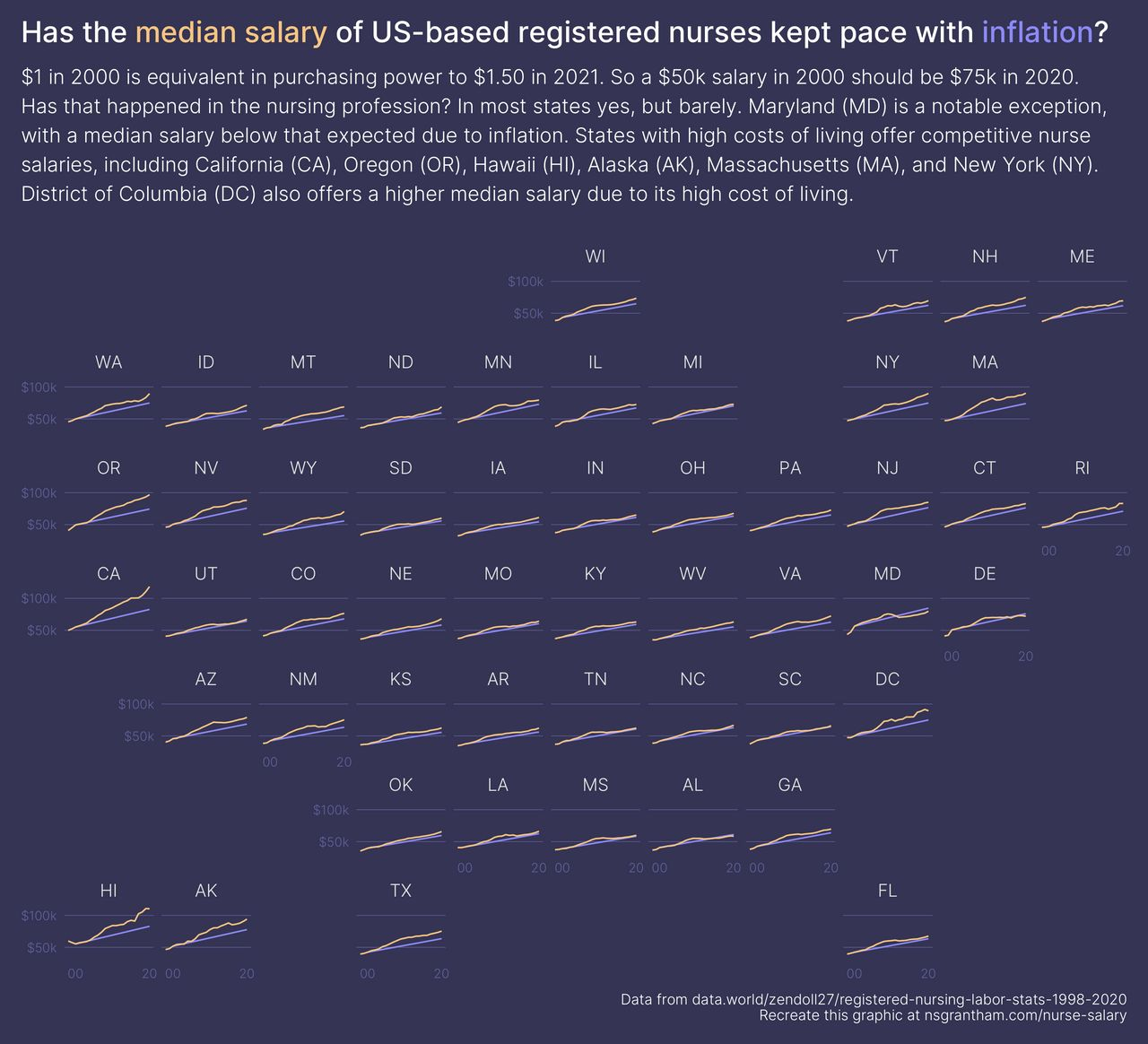# Nurse Salaries and Inflation

October 07, 2021
Medium: R and ggplot2
Large: JPEG
``````library(tidyverse)  # 1.3.1
library(geofacet)   # 0.2.0
library(ggtext)     # 0.1.1
library(ggdark)     # 0.2.1
library(glue)       # 1.4.2

states <- set_names(c(state.abb, "DC"), nm = c(state.name, "District of Columbia"))

select(
year = Year,
state = State,
salary = `Annual Salary Median`
) %>%
mutate(state = recode(state, !!! states)) %>%
filter(state %in% states) %>%
mutate(type = "salary")

inflation <- nurses %>%
select(year, state, salary) %>%
filter(year == 2000, state %in% states) %>%
pivot_wider(names_from = year, values_from = salary) %>%
mutate(`2020` = 1.5 * `2000`) %>%
pivot_longer(`2000`:`2020`, names_to = "year", values_to = "salary") %>%
mutate(
year = as.numeric(year),
type = "inflation"
)

salary_color <- "#f8c582"
inflation_color <- "#8d8af8"

bind_rows(
nurses,
inflation
) %>%
ggplot(aes(year, salary, group = type, color = type)) +
geom_line() +
facet_geo(~ state) +
scale_color_manual(values = c(inflation_color, salary_color)) +
scale_y_continuous(breaks = c(50000, 100000), labels = c(" \$50k", "\$100k")) +
scale_x_continuous(breaks = c(2000, 2020), labels = c("00", "20")) +
labs(
title = glue("Has the <span style='color:{salary_color}'>median salary</span> of US-based registered nurses kept pace with <span style='color:{inflation_color}'>inflation</span>?"),
subtitle = "\$1 in 2000 is equivalent in purchasing power to \$1.50 in 2021. So a \$50k salary in 2000 should be \$75k in 2020.\nHas that happened in the nursing profession? In most states yes, but barely. Maryland (MD) is a notable exception,\nwith a median salary below that expected due to inflation. States with high costs of living offer competitive nurse\nsalaries, including California (CA), Oregon (OR), Hawaii (HI), Alaska (AK), Massachusetts (MA), and New York (NY).\nDistrict of Columbia (DC) also offers a higher median salary due to its high cost of living.",
caption = "Data from data.world/zendoll27/registered-nursing-labor-stats-1998-2020\nRecreate this graphic at nsgrantham.com/nurse-salary",
x = NULL,
y = NULL
) +
guides(label = NULL, color = "none") +
dark_theme_minimal(base_family = "Inter-Light") +
theme(
plot.background = element_rect(color = "#353454", fill = "#353454"),
plot.margin = margin(1, 1, 1, 1, unit = "line"),
plot.title = element_markdown(family = "Inter-Medium", size = 20, margin = margin(0, 0, 1, 0, unit = "line")),
plot.subtitle = element_text(size = 14, lineheight = 1.2, margin = margin(0, 0, 1, 0, unit = "line")),
plot.title.position = "plot",
plot.caption = element_text(size = 10, margin = margin(1, 0, 0, 0, unit = "line")),
strip.text = element_text(size = 12, margin = margin(1, 0, 0, 0, unit = "line")),
panel.grid.major.y = element_line(color = "#62619D", size = 0.2),
panel.grid.major.x = element_blank(),
panel.grid.minor.x = element_blank(),
panel.grid.minor.y = element_blank(),
axis.text.y = element_text(color = "#62619D"),
axis.text.x = element_text(color = "#62619D")
)

ggsave("nurse-salary.png", width = 11, height = 10)
``````